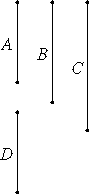# Proposition 25

If two numbers are relatively prime, then the product of one of them with itself is relatively prime to the remaining one.

Let A and B be two numbers relatively prime, and let A multiplied by itself make C.I say that B and C are relatively prime.

Make D equal to A.

VII.24

Since A and B are relatively prime, and A equals D, therefore D and B are also relatively prime. Therefore each of the two numbers D and A is relatively prime to B. Therefore the product of D and A is also relatively prime to B.

But the number which is the product of D and A is C. Therefore C and B are relatively prime.

Therefore, if two numbers are relatively prime, then the product of one of them with itself is relatively prime to the remaining one.

Q.E.D.

## Guide

This proposition says that if a is relatively prime to b, then a2 is also relatively prime to b.

It’s a special case of the previous proposition and hardly needs its own enunciation. It is used in VII.27 and IX.15.